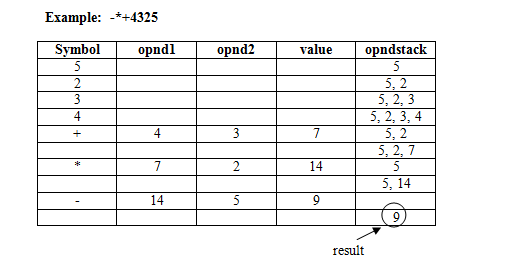# Evaluation of Prefix expression

## Application of Stacks

Pro Developer`s Tip: Experience the power of cloud by migrating your programming tools such as emulators and IDE`s into the cloud and access it remotely from anywhere, anytime at your convenience on any device (PC / Mac / Linux / android / iOS) with high performance hosted citrix vdi from CloudDesktopOnline.  Looking for a reliable cloud platform for your enterprise?  Know more about MS Azure and managed azure services feel free to visit one of the leading cloud hosting providers – Apps4Rent.

### Evaluation of Prefix expression

The algorithm for evaluating a prefix expression is as follows:

• Accept a prefix string from the user.

say (-*+ABCD), let A=4, B=3, C=2, D=5

i.e. (-*+4325) is the input prefix string.

• Start scanning the string from the right one character at a time.
• If it is an operand, push it in stack.
• If it is an operator, pop opnd1, opnd2 and perform the operation, specified by the operator.  Push the result in the stack.
• Repeat these steps until arr of input prefix strings ends.This algorithm works fine, if given prefix expressions are evaluated from left to right without considering precedence of operators, which will not give correct result in all the cases, where precedence rules of operators must be followed to get correct result.

For example:  Consider the following two prefix notations:

(a)    +*435  and  (b)  *+435

Infix equivalent of a is 4*3+5 and that of b is 4+3*5.  Result of a is 12+5=17 and that of b is 7*5=35, which is not the desired result since the precedence of * operator is higher as compared to +.  Result should be 4+3*5=4+15 = 19 or if the expression is (4+3)*5, then (7)*5=7*5=35.

Thus precedence of operators and availability of brackets must be kept in mind for evaluating a given prefix string to result in a correct output.

Therefore, correct result may only be achieved from a prefix string, if while converting from infix to prefix, due consideration is kept in mind for precedence of operators and that of brackets.

Program to evaluate Prefix expression:

#include <stdio.h>
#include <conio.h>
#include <stdlib.h>
#include <math.h>

#define MAX 80

struct stack
{int top;
double items[MAX];
};

void push(struct stack *,int);
int pop(struct stack *);
void display(struct stack *);
int isdigit(char);
double eval(char []);
double oper(char,double,double);

void push(struct stack *s,int x)
{
if(s->top==MAX-1)
{printf(“nStack Overflow…!”);
return;
}
else
{s->items[++s->top]=x;
}
}

int pop(struct stack *s)
{
if(s->top==-1)
{printf(“nStack Underflow…!”);
return 0;
}
else
{return(s->items[s->top–]);
}
}

void display(struct stack *s)
{
int ctr;
if(s->top==-1)
{printf(“nStack is empty…!”);
return;
}
else
{
ctr=s->top;
while(ctr!=-1)
printf(“%dt”,s->items[ctr–]);
}
}

int isdigit(char ch)
{
return(ch>=’0′ && ch<=’9′);
}

double oper(char c,double opnd1,double opnd2)
{
switch(c)
{
case ‘+’: return(opnd1+opnd2);
case ‘-‘: return(opnd1-opnd2);
case ‘*’: return(opnd1*opnd2);
case ‘/’: return(opnd1/opnd2);
case ‘^’: return(pow(opnd1,opnd2));
default: printf(“nInvalid operator…!”);return 0;
}
}

double eval(char str[])
{
char c;
double opnd1,opnd2,val;
struct stack stk;
stk.top=-1;
int i;
for(i=0;(c=str[i])!=’�’;i++)
{
if(isdigit(c))
push(&stk,(double)(c-‘0′));
else
{
opnd2=pop(&stk);
opnd1=pop(&stk);
val=oper(c,opnd1,opnd2);
push(&stk,val);
}
}
return(pop(&stk));
}

void main()
{
char str[MAX];
int i,j,k;
char temp;
clrscr();
printf(“ntEnter prefix string:”);
for(i=0;(str[i]=getchar())!=’n’;i++);
str[i]=’�’;
k=i;
printf(“nThe prefix expression is: %s”,str);
for(i=0,j=k-1;i<=j;i++,j–)
{
temp=str[i];
str[i]=str[j];
str[j]=temp;
}
printf(“nPrefix expression after reversing is %s”,str);
printf(“nResult after evaluation is: %f”,eval(str));
getch();
}